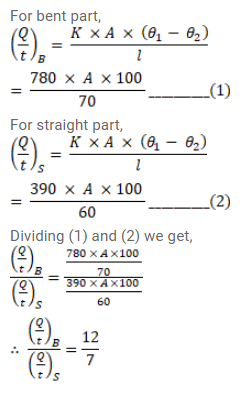# Suppose the bent part of the frame of the previous problemQuestion:

Suppose the bent part of the frame of the previous problem has a thermal conductivity of $780 \mathrm{~J} / \mathrm{m}-\mathrm{s}^{\circ} \mathrm{C}$ whereas it is $390 \mathrm{~J} / \mathrm{m}-\mathrm{s}-{ }^{\circ} \mathrm{C}$ for the straight part. Calculate the ratio of the rate of heat flow through the bent part to the rate of heat flow through the straight part.

Solution: